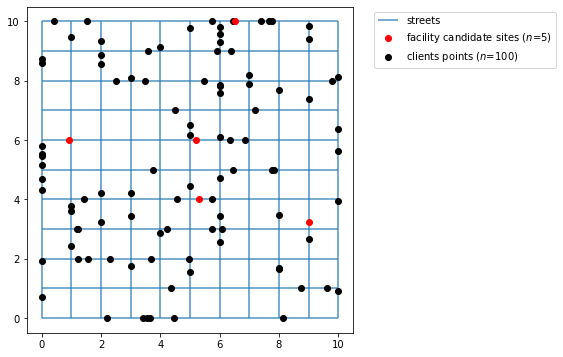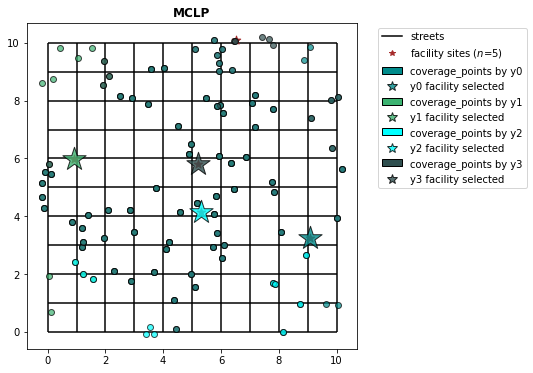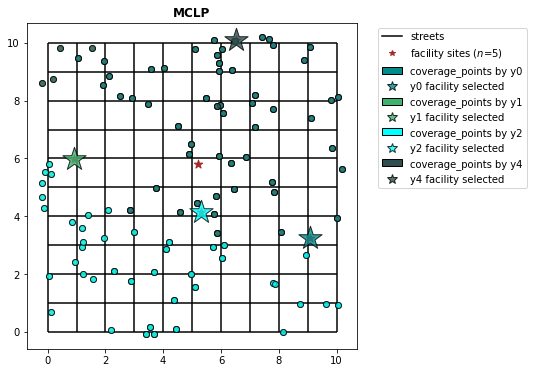# Maximal Coverage Location Problem¶

LSCP try to minimize the amount of facilities candidate sites in a maximum service standard but then arise another problem: the budget. Sometimes it requires many facilities sites to reach a complete coverage, and there are circumstances when the resources are not available and it's plausible to know how much coverage we can reach using a exact number of facilities. MCLP class try to solve this problem:

Maximize the amount of demand covered within a maximal service distance or time standard by locating a fixed number of facilities

MCLP in math notation:

$\begin{array} \displaystyle \textbf{Maximize} & \sum_{i=1}^{n}{a_iy_i} && (1) \\ \displaystyle \textbf{Subject to:} & \sum_{j\in N_i}{x_j \geq y_i} & \forall i & (2) \\ & \sum_{j}{x_j = p} & \forall j & (3) \\ & y_i \in \{0,1\} & \forall i & (4) \\ & x_j \in \{0,1\} & \forall j & (5) \\ \end{array}$

$\begin{array} \displaystyle \textbf{Where:}\\ & & \displaystyle i & \small = & \textrm{index referencing nodes of the network as demand} \\ & & j & \small = & \textrm{index referencing nodes of the network as potential facility sites} \\ & & S & \small = & \textrm{maximal acceptable service distance or time standard} \\ & & d_{ij} & \small = & \textrm{shortest distance or travel time between nodes} i \textrm{and} j \\ & & N_i & \small = & \{j | d_{ij} < S\} \\ & & p & \small = & \textrm{number of facilities to be located} \\ & & x_j & \small = & \begin{cases} 1, \text{if a facility is located at node } j \\ 0, \text{otherwise} \\ \end{cases} \\ & & y_i & \small = & \begin{cases} 1, \textrm{if demand } i \textrm{ is covered within a service standard} \\ 0, \textrm{otherwise} \\ \end{cases}\end{array}$

This excerpt above was quoted from Church L., Murray, A. (2018)

This tutorial solves MCLP using spopt.locate.coverage.MCLP instance that depends on a array 2D representing the costs between facilities candidate sites and demand points. For that it uses a lattice 10x10 with simulated points to calculate the costs.

In :
from spopt.locate.coverage import MCLP
from spopt.locate.util import simulated_geo_points

import numpy
import geopandas
import pulp
import spaghetti
from shapely.geometry import Point
import matplotlib.pyplot as plt


Since the model needs a distance cost matrix we should define some variables. In the comments, it's defined what these variables are for but solver. The solver, assigned below as pulp.PULP_CBC_CMD, is an interface to optimization solver developed by COIN-OR. If you want to use another optimization interface as Gurobi or CPLEX see this guide that explains how to achieve this.

In :
CLIENT_COUNT = 100 # quantity demand points
FACILITY_COUNT = 5 # quantity supply points

MAX_COVERAGE = 7 # maximum service radius
P_FACILITIES = 4

# Random seeds for reproducibility
CLIENT_SEED = 5
FACILITY_SEED = 6

solver = pulp.PULP_CBC_CMD(msg=False) # see solvers available in pulp reference


## Lattice 10x10¶

Create lattice 10x10 with 9 vertical lines in interior.

In :
lattice = spaghetti.regular_lattice((0, 0, 10, 10), 9, exterior=True)
ntw = spaghetti.Network(in_data=lattice)


Transform spaghetti instance to geopandas geodataframe.

In :
street = spaghetti.element_as_gdf(ntw, arcs=True)

street_buffered = geopandas.GeoDataFrame(
geopandas.GeoSeries(street["geometry"].buffer(0.2).unary_union),
crs=street.crs,
columns=["geometry"],
)


Plotting the network created by spaghetti we can verify that it seems a district with quarters and streets.

In :
street.plot()

Out:
<AxesSubplot:>

## Simulate points in a network¶

The function simulated_geo_points simulates points inside a network. In this case, it uses a lattice network 10x10 created by using spaghetti package. Below we use the function defined above and simulate the points inside lattice bounds.

In :
client_points = simulated_geo_points(street_buffered, needed=CLIENT_COUNT, seed=CLIENT_SEED)
facility_points = simulated_geo_points(
street_buffered, needed=FACILITY_COUNT, seed=FACILITY_SEED
)


Plotting the 100 client and 5 facility points we can see that the function generates dummy points to an area of 10x10 which is the area created by our lattice created on previous cells.

In :
fig, ax = plt.subplots(figsize=(6, 6))
street.plot(ax=ax, alpha=0.8, zorder=1, label='streets')
facility_points.plot(ax=ax, color='red', zorder=2, label='facility candidate sites ($n$=5)')
client_points.plot(ax=ax, color='black', label='clients points ($n$=100)')
plt.legend(loc='upper left', bbox_to_anchor=(1.05, 1))

Out:
<matplotlib.legend.Legend at 0x7fd461da6370>

Here, for each client point the model suppose that there is a weight. So, we use randint function from numpy to also simulate these weights.

In :
ai = numpy.random.randint(1, 12, CLIENT_COUNT)


The weight is simulate with a 1-12 range, the minimum is 1 and the maximum is 12.

In :
ai

Out:
array([10,  7,  3,  6,  6,  2,  5,  6,  1,  3,  3,  4,  6, 10,  6,  3, 11,
8,  9,  6,  7, 11, 11, 11,  4,  6,  2,  3,  4,  7, 10, 10,  9,  1,
11,  4,  8,  5,  9,  2,  3,  5,  2,  6,  6,  7,  2, 10,  1,  6, 11,
9, 10,  2,  3,  3, 10,  6, 11,  5,  2,  7,  4,  2,  5, 10,  9, 11,
11, 11,  5,  7,  3, 10,  7,  3,  6,  3,  8,  6,  2,  2,  8,  6,  5,
3,  9,  9,  8,  9,  1, 11,  9, 10,  3,  1,  8,  7,  1,  8])

## Transform simulated points to real points¶

To use cost matrix or geodataframes we have to pay attention in some details. The client and facility points simulated don't belong to network, so if we calculate the distances now we are supposed to receive a wrong result. Before calculating distances we snap points to the networok and then calculate the distances.

Below we snap points that is not spatially belong to network and create new real points geodataframes.

In :
ntw.snapobservations(client_points, "clients", attribute=True)
clients_snapped = spaghetti.element_as_gdf(
ntw, pp_name="clients", snapped=True
)

ntw.snapobservations(facility_points, "facilities", attribute=True)
facilities_snapped = spaghetti.element_as_gdf(
ntw, pp_name="facilities", snapped=True
)


Now the plot seems more organized as the points belong to network. The network created is plotted below with facility points and clients points:

In :
fig, ax = plt.subplots(figsize=(6, 6))
street.plot(ax=ax, alpha=0.8, zorder=1, label='streets')
facilities_snapped.plot(ax=ax, color='red', zorder=2, label='facility candidate sites ($n$=5)')
clients_snapped.plot(ax=ax, color='black', label='clients points ($n$=100)')
plt.legend(loc='upper left', bbox_to_anchor=(1.05, 1))

Out:
<matplotlib.legend.Legend at 0x7f7784ec7f10>## Calculating the cost matrix¶

Calculate distance between clients and facilities.

In :
cost_matrix = ntw.allneighbordistances(
sourcepattern=ntw.pointpatterns["clients"],
destpattern=ntw.pointpatterns["facilities"],
)


The expected result here is a Dijkstra distance between clients and facilities points, so we our case an array 2D 100x5.

In :
cost_matrix

Out:
array([[12.60302601,  3.93598651,  8.16571655,  6.04319467,  5.65607701],
[13.10096347,  4.43392397,  8.66365401,  6.54113213,  5.15813955],
[ 6.9095462 ,  4.2425067 ,  2.47223674,  0.34971486,  5.34955682],
[ 2.98196832,  7.84581224,  3.45534114,  3.57786302,  6.25374871],
[ 7.5002892 ,  6.32806975,  4.55779979,  6.43527791, 11.75939222],
[ 0.60209077, 11.42987132,  5.03940023,  7.16192211,  9.8378078 ],
[ 5.37335867,  6.20113923,  2.43086927,  4.30834738,  9.6324617 ],
[ 5.40801577,  5.41976478,  3.02929369,  1.15181557,  4.85108725],
[ 3.68807115,  8.51585171,  2.12538061,  4.24790249,  7.94717417],
[14.22503627,  4.60274429,  9.78772681,  7.66520493,  4.98931924],
[10.32521229,  4.99225179,  7.38272288,  9.260201  , 14.58431531],
[ 6.65436171,  7.98732222,  5.59685112,  3.719373  ,  2.58135531],
[11.55510375,  1.11193575,  7.11779429,  5.37988496, 10.70399927],
[10.90832519,  1.75871431,  6.47101573,  6.02666352, 11.35077783],
[ 9.29354019,  9.53424036,  7.14376926,  5.26629115,  0.05782317],
[11.25279502,  3.57498553,  6.81548556,  4.69296368,  6.01707799],
[ 6.14400601, 11.47696651,  9.08649542,  7.2090173 ,  3.09171102],
[10.43008909,  2.23695041,  5.99277963,  6.50489962, 11.82901393],
[ 1.79838406, 11.13134457,  4.74087347,  6.86339535,  9.53928104],
[ 2.93052752,  7.89725303,  3.50678194,  3.62930382,  6.30518951],
[11.55272282,  6.21976231,  8.61023341, 10.48771153, 15.81182584],
[ 8.83964081,  3.66742137,  5.89715141,  7.77462952, 13.09874384],
[ 4.11777697,  9.45073748,  7.06026638,  5.18278826,  7.11794005],
[ 8.69768642,  8.63527408,  5.75519701,  7.63267513, 12.95678945],
[ 8.2652832 ,  6.56249735,  4.79222739,  2.66970551,  3.02956617],
[ 1.71437731,  9.6185832 ,  3.2281121 ,  5.35063398,  8.02651967],
[ 4.30308213,  6.52469842,  2.13422733,  2.25674921,  5.95602089],
[ 9.31612329,  8.64908379,  6.25861269,  4.38113458,  0.94297974],
[ 2.86540683, 13.69318738,  7.30271629,  9.42523817, 12.10112386],
[ 8.95995574,  2.29291624,  4.52264628,  6.4001244 , 11.72423871],
[10.54288208,  7.87584258,  6.10557262,  3.98305074,  1.71622094],
[ 8.58885878,  8.74410173,  5.64636937,  7.52384749, 12.8479618 ],
[ 2.51163835, 12.82132215,  6.43085106,  8.55337294, 11.22925863],
[ 5.19213144,  5.63564912,  0.75482198,  1.74285727,  7.06697159],
[ 4.1276352 , 13.2053253 ,  6.81485421,  8.93737609, 11.61326178],
[ 3.99217608,  6.83560448,  0.44513338,  2.94281263,  6.75645905],
[ 5.88198594, 11.21494644,  8.82447535,  6.94699723,  5.35373109],
[ 8.24225403,  4.58552653,  3.80494457,  1.68242269,  5.006537  ],
[10.89255004,  6.22551054,  6.45524058,  4.3327187 ,  3.36655299],
[ 6.58504851, 11.91800902,  9.52753792,  7.6500598 ,  2.65066851],
[ 5.44204086,  8.77500136,  6.38453026,  4.50705215,  3.79367617],
[ 5.56289993,  7.26488062,  4.87440953,  2.99693141,  3.6728171 ],
[ 7.96716366, 10.86061689,  8.4701458 ,  6.59266768,  1.26855337],
[ 7.9603294 ,  5.3726311 ,  5.01783999,  6.89531811, 12.21943243],
[ 8.68198919,  4.65097132,  5.73949978,  7.6169779 , 12.94109221],
[ 9.06064716,  8.39360767,  6.00313657,  4.12565845,  1.19845586],
[15.325265  ,  4.65822551, 10.88795554,  8.76543366,  6.08954798],
[ 3.51444772,  7.81851278,  1.95175718,  3.92572094,  7.77355074],
[ 3.33469883, 14.16247938,  7.77200828,  9.89453017, 12.57041585],
[ 4.46482284,  6.36295772,  1.40731225,  2.0950085 ,  5.79428018],
[11.20742649,  1.459613  ,  6.77011704,  5.72756222, 11.05167653],
[11.15442417,  5.67335639,  6.71711471,  4.59459283,  3.91870714],
[ 5.17021584,  5.65756471,  0.73290638,  2.6103845 ,  7.93449881],
[ 5.54400588, 10.87696639,  8.48649529,  6.60901717,  5.28490286],
[ 5.28695668,  8.04600382,  2.34446727,  4.22194539,  9.5460597 ],
[ 7.33259845,  6.66555896,  4.27508786,  2.39760974,  2.92650457],
[ 8.08642618, 10.74135437,  8.35088328,  6.47340516,  1.14929085],
[ 7.97403829,  2.85374226,  3.53672884,  4.96095042, 10.28506473],
[ 5.04455411,  6.2884064 ,  2.1020647 ,  3.97954282,  9.30365713],
[ 8.05520721,  3.2777533 ,  5.1127178 ,  6.99019592, 12.31431023],
[ 8.033197  ,  3.2997635 ,  5.09070759,  6.96818571, 12.29230002],
[ 4.88391014,  5.94387041,  3.55339931,  1.6759212 ,  4.62480712],
[ 3.38092176,  9.44685879,  6.32341117,  5.17890958,  7.85479527],
[ 5.83945489,  5.17241539,  2.78194429,  0.90446618,  4.41964814],
[10.25764123,  4.57013932,  5.82033178,  3.69780989,  5.02192421],
[ 3.16471551,  8.168245  ,  1.7777739 ,  3.90029578,  7.59956747],
[ 8.83620663,  8.49675387,  5.89371722,  7.77119534, 13.09530965],
[ 7.60754658,  6.94050708,  4.55003599,  2.67255787,  2.65155644],
[ 4.14555919,  9.4785197 ,  7.0880486 ,  5.21057048,  5.09015784],
[ 7.24126831,  4.57422881,  2.80395885,  0.68143697,  5.01783472],
[ 5.70322513,  8.53100569,  2.76073572,  4.63821384,  9.96232815],
[ 9.27617639,  9.55160416,  7.16113307,  5.28365495,  0.04045936],
[ 2.5651854 , 11.39296595,  5.00249486,  7.12501674,  9.80090243],
[14.22296519,  3.5559257 ,  9.78565573,  7.66313385,  6.03613783],
[ 8.33806089,  2.48971967,  3.90075143,  5.77822955, 11.10234386],
[14.34079531,  3.51301476,  9.90348585,  7.78096397,  7.91830771],
[ 7.55811406,  6.89107456,  4.50060346,  2.62312535,  2.70098897],
[ 9.54667188,  8.87963238,  6.48916129,  4.61168317,  0.71243114],
[ 6.99771477,  3.83006578,  2.56040532,  2.43788343,  7.76199775],
[10.85478728,  4.18774778,  6.41747782,  4.29495594,  5.40431574],
[ 6.89563349,  8.43732701,  3.95314408,  5.8306222 , 11.15473651],
[12.29945454,  3.63241504,  7.86214508,  5.7396232 ,  5.95964848],
[ 6.57929244,  6.75366806,  3.63680304,  5.51428115, 10.83839547],
[ 8.35675866,  8.47102189,  6.0805508 ,  4.20307268,  1.90234436],
[11.26183   ,  1.40520949,  6.82452055,  5.67315871, 10.99727302],
[ 6.92663397,  8.25959447,  5.86912337,  3.99164526,  2.30908306],
[ 6.97410775,  3.8536728 ,  2.53679829,  3.96088096,  9.28499527],
[10.00715257,  8.82062799,  6.94964198,  4.92783614,  0.77143554],
[ 8.83013405,  7.9976465 ,  5.77262346,  3.89514534,  1.59441703],
[ 6.69445759,  4.63850292,  2.86823295,  4.74571107, 10.06982539],
[ 2.60649588, 11.43427644,  5.04380534,  7.16632722,  9.84221291],
[ 9.01806225,  4.31489826,  6.07557284,  7.95305096, 13.27716527],
[ 7.49191577,  3.84104474,  4.07077477,  5.94825289, 11.2723672 ],
[ 7.80056437,  9.53239613,  4.85807497,  6.73555308, 12.0596674 ],
[ 8.85156915,  2.48139135,  4.71112139,  6.58859951, 11.91271382],
[10.04988811,  2.61715138,  5.61257866,  6.8851006 , 12.20921491],
[ 3.68039673,  7.65256378,  1.26209268,  3.38461456,  7.08388625],
[10.04984807,  7.28311243,  7.10735867,  8.98483678, 14.3089511 ],
[ 8.34309643, 10.48468413,  8.09421303,  6.21673491,  0.8926206 ],
[14.48203148,  3.65425093, 10.04472202,  7.92220014,  7.77707154]])

With MCLP.from_cost_matrix we model the MCL problem to cover all demand points with $p$ facility points within a max_coverage meters as service radius using cost matrix calculated previously.

In :
mclp_from_cost_matrix = MCLP.from_cost_matrix(cost_matrix, ai, MAX_COVERAGE, p_facilities=P_FACILITIES)
result = mclp_from_cost_matrix.solve(solver)


Expected result is an instance of MCLP.

In :
mclp_from_cost_matrix

Out:
<spopt.locate.coverage.MCLP at 0x7f7785f9d130>

## Using GeoDataFrame¶

Assigning service load array to demand geodataframe

In :
clients_snapped['weights'] = ai

In :
clients_snapped

Out:
id geometry comp_label weights
0 0 POINT (2.00000 8.85562) 0 10
1 1 POINT (2.00000 9.35355) 0 7
2 2 POINT (5.00000 6.16214) 0 3
3 3 POINT (7.76544 5.00000) 0 6
4 4 POINT (3.00000 1.75230) 0 6
... ... ... ... ...
95 95 POINT (0.00000 4.30248) 0 1
96 96 POINT (6.00000 3.42781) 0 8
97 97 POINT (2.20274 0.00000) 0 7
98 98 POINT (7.40431 10.00000) 0 1
99 99 POINT (0.00000 8.73462) 0 8

100 rows × 4 columns

With MCLP.from_geodataframe we model the MCL problem to cover all demand points with $p$ facility points within a max_coverage meters as service radius using geodataframes without calculating the cost matrix previously.

In :
mclp_from_geodataframe = MCLP.from_geodataframe(
clients_snapped,
facilities_snapped,
"geometry",
"geometry",
"weights",
MAX_COVERAGE,
p_facilities=P_FACILITIES,
distance_metric="euclidean"
)
mclp_from_geodataframe = mclp_from_geodataframe.solve(solver)


Expected result is an instance of MCLP.

In :
mclp_from_geodataframe

Out:
<spopt.locate.coverage.MCLP at 0x7f778487d430>

## Plotting the results¶

The cell below describe the plotting of the results. For each method from MCLP class (from_cost_matrix, from_geodataframe) there is a plot displaying the facility site that was selected with a star colored and the points covered with the same color. Sometimes the demand points will be colored with not expected colors, it represents the coverage overlapping.

In :
from matplotlib.patches import Patch
import matplotlib.lines as mlines

dv_colors = [
"darkcyan",
"mediumseagreen",
"cyan",
"darkslategray",
"lightskyblue",
"limegreen",
"darkgoldenrod",
"peachpuff",
"coral",
"mediumvioletred",
"blueviolet",
"fuchsia",
"thistle",
"lavender",
]

def plot_results(model, facility_points):
arr_points = []
fac_sites = []

for i in range(FACILITY_COUNT):
if model.fac2cli[i]:

geom = client_points.iloc[model.fac2cli[i]]['geometry']
arr_points.append(geom)
fac_sites.append(i)

fig, ax = plt.subplots(figsize=(6, 6))
legend_elements = []

street.plot(ax=ax, alpha=1, color='black', zorder=1)
legend_elements.append(mlines.Line2D(
[],
[],
color='black',
label='streets',
))

facility_points.plot(ax=ax, color='brown', marker="*", markersize=80, zorder=2)
legend_elements.append(mlines.Line2D(
[],
[],
color='brown',
marker="*",
linewidth=0,
label=f'facility sites ($n$={FACILITY_COUNT})'
))

for i in range(len(arr_points)):
gdf = geopandas.GeoDataFrame(arr_points[i])

label = f"coverage_points by y{fac_sites[i]}"
legend_elements.append(Patch(facecolor=dv_colors[i], edgecolor="k", label=label))

gdf.plot(ax=ax, zorder=3, alpha=0.7, edgecolor="k", color=dv_colors[i], label=label)
facility_points.iloc[[fac_sites[i]]].plot(ax=ax,
marker="*",
markersize=200 * 3.0,
alpha=0.8,
zorder=4,
edgecolor="k",
facecolor=dv_colors[i])

legend_elements.append(mlines.Line2D(
[],
[],
color=dv_colors[i],
marker="*",
ms=20 / 2,
markeredgecolor="k",
linewidth=0,
alpha=0.8,
label=f"y{fac_sites[i]} facility selected",
))

plt.title("MCLP", fontweight="bold")
plt.legend(handles = legend_elements, loc='upper left', bbox_to_anchor=(1.05, 1))


### MCLP built from cost matrix¶

In :
mclp_from_cost_matrix.facility_client_array()
plot_results(mclp_from_cost_matrix, facility_points)### MCLP built from geodataframes¶

In :
mclp_from_geodataframe.facility_client_array()
plot_results(mclp_from_geodataframe, facility_points)You may notice that the models are different. This result is expected as the distance between facility and demand points is calculated with different metrics. The cost matrix is calculated with dijkstra distance while the distance using geodataframe is calculated with euclidean distance.